# Learning Python

## Programming Basics

### Programming is Good

• Learn how to control your best tool: computer
• Learn to think logically
• Learn how to solve big problem
• Our future civilization depends on it

### You Will Program

You may not acknowledge it,
but everyone will program:

### Some Programs are More Complicated

Spreadsheets can be incredibly complex:### What is programming?

A bit of a cooking recipe for computers

```      • Crack an egg into a bowl
• Whisk the egg
• Put a pan on medium heat
• Grease the pan
• Pour the eggs into the pan
```

### What is programming?

• Receive input values• Transform values through logic• Send output values### What is programming robots?

• Receive input values: Sensors, Camera Images, Joysticks
• Transform values through logic
• Send output values: Actuators, Motors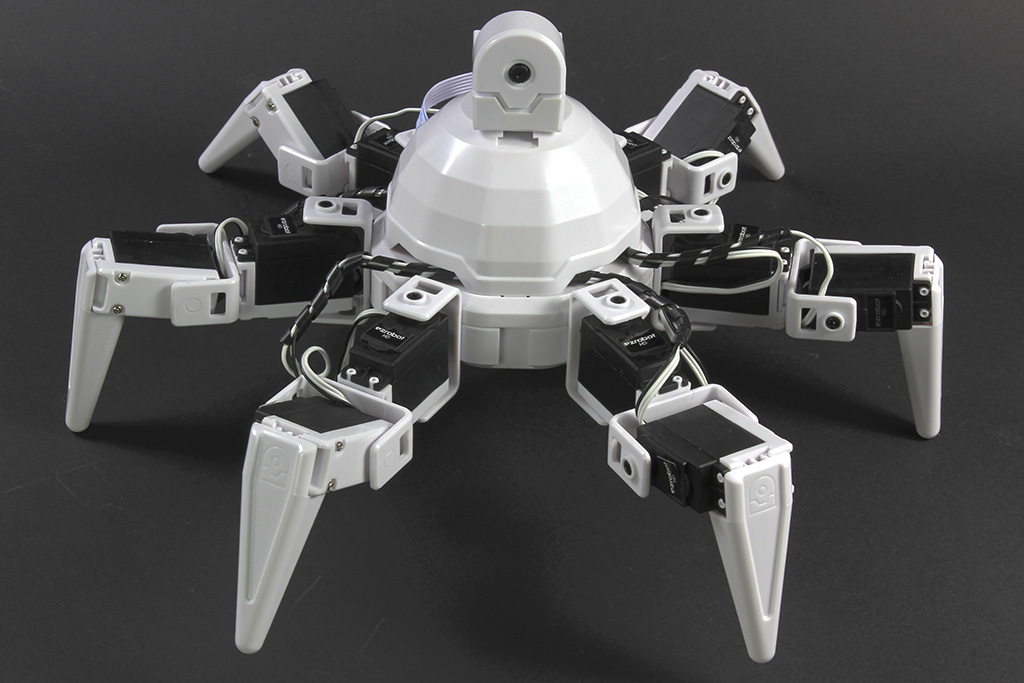### What does programming look like?

Have you programmed in Scratch?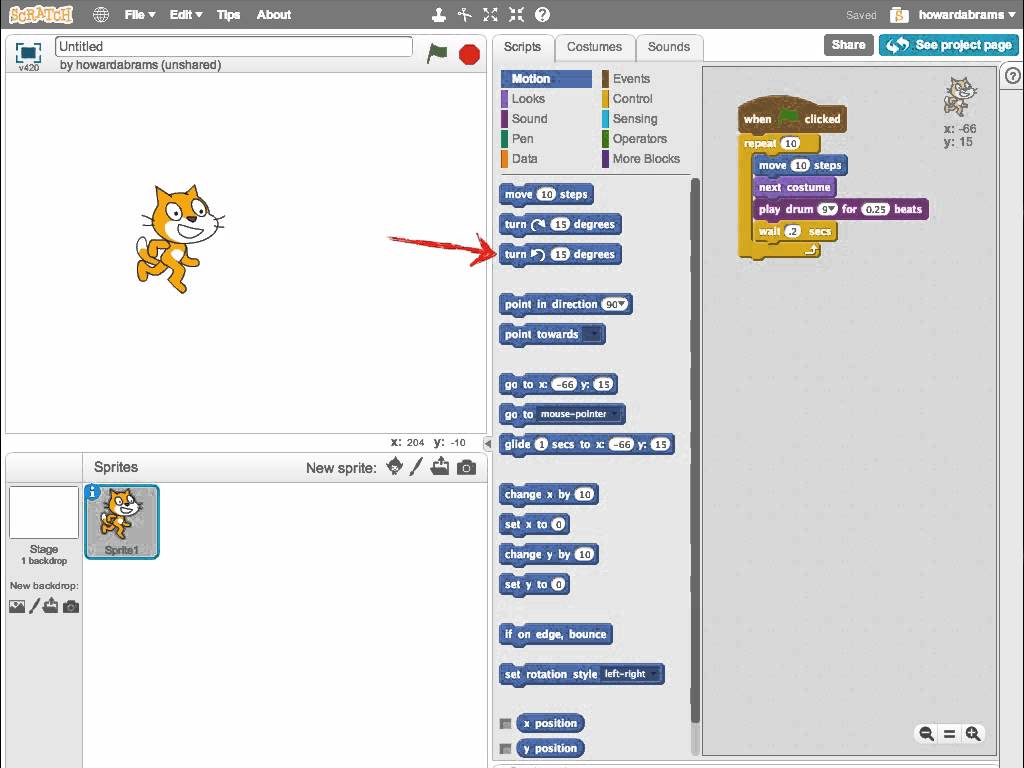### What does programming look like?

Unlike Scratch, non-graphical programs have syntax:

```     public int[] increment(int[] array) {
int[] newarray = new int[array.length];
for (int i = 0; i < array.length; i++) {
newarray[i] = array[i] + 1;
}
return newarray;
}
```

This creates a function, `increment`, takes a list of numbers, and returns a list with each number one larger.

This language is called Java.

### What does programming look like?

The previous code written in another language:

```     increment :: [Int] -> [Int]
increment = map (1+)
```

And yet another language:

```     (defn increment [array]
(map #(inc %) array))
```

Often look different, but generally do the same thing.

### Is it Programming?

If you swear at it, it is programming.Remember: We improve only during the struggle.
Just don't take it out on your teammates! 😉

## Programming Python

• We Will Program in Python

### Why Python?

• Easy to write, read and understand
• Easy to get started and learn
• Very flexible, yet powerful
• Lots and lots of libraries
• Large community

### What can Python Do?

Created in late 1980s for beginning programmers,
it is now used everywhere.

• Intel uses it to test microchips
• Instagram uses it for most of their code
• I use it at Workday to create thousands of virtual computers
• We can use it to program our robots### What’s Python Like?

Simple syntax. Let’s create a variable:

```     the_answer = 42
```

Conditions pay attention to spaces:

```     if the_answer == 42:
return "Yes"
else:
return "No"
```

### What’s Python Like?

Remember that big ugly Java function?
Here is a Python version:

```    def increment(array):
return [i + 1 for i in array]
```

To run it:

```    increment( [1, 10, 41, -3] )
```

Returns:

```    [2, 11, 42, -2]
```

### Python Demo Time

Pull out your calculator, and type: 2100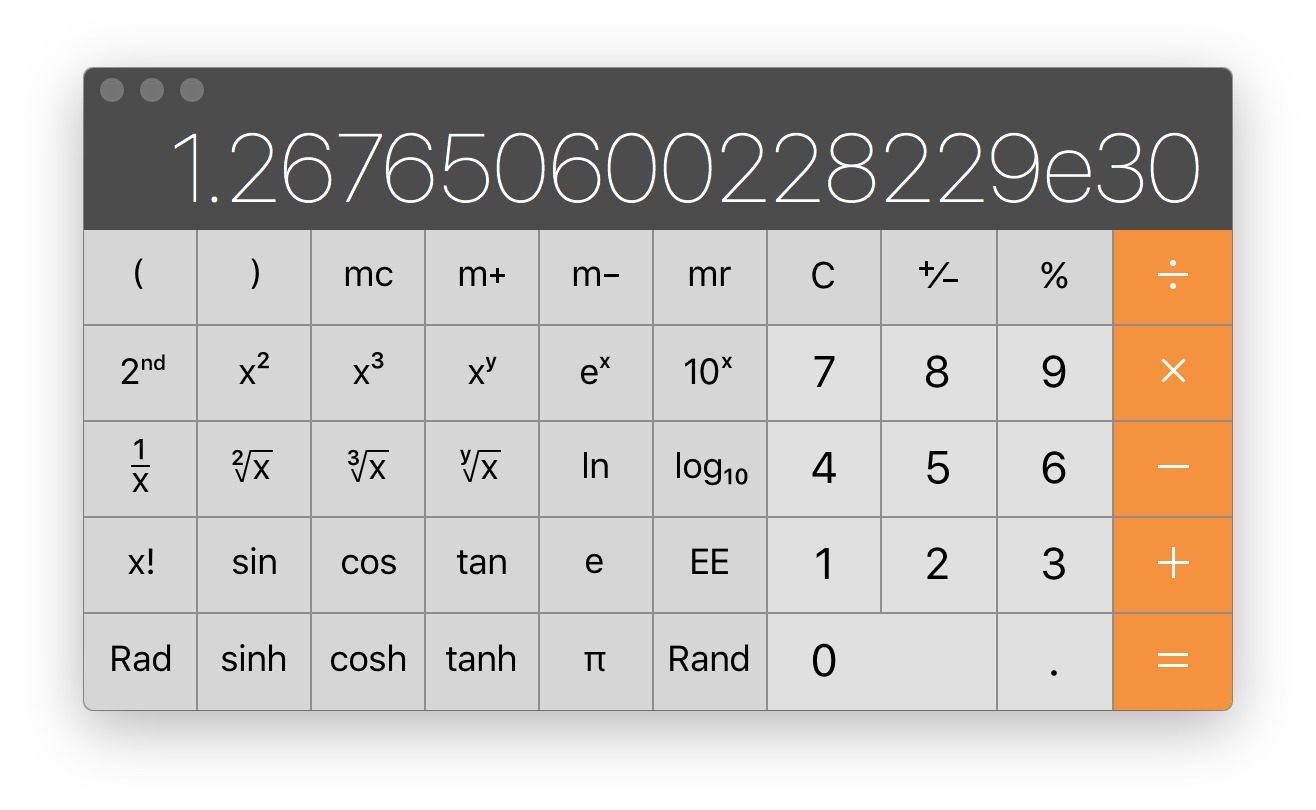### Python Demo Time

Let’s start `python` and type the equation:

```     2**100
```

Python returns:

```   1267650600228229401496703205376
```

### Python Demo Time

Try again on your calculator: 21000Most calculators can’t even handle the number.

### Python Demo Time

Try this equation in Python:

```     2**1000
```

Python returns:

```   10715086071862673209484250490600018105614048117055336074437503883703510511249361224931983788156958581275946729175531468251871452856923140435984577574698574803934567774824230985421074605062371141877954182153046474983581941267398767559165543946077062914571196477686542167660429831652624386837205668069376
```

## Learning Python

You need to learn Python…fast!

We don’t have time for weekly lessons from a mentor.

You need to teach yourself as much as you can.
(Mentors will still help, though).

“For the things we have to learn before we can do them, we learn by doing them.”  — Aristotle

### We Can’t Spoon Feed You!

Sorry. This is College Prep work.

### How Do You Learn?

Everyone learned differently.

Let me show you ways to learn Python

### Online Tutorials

Lots of free web tutorials for Python.
The problem is choosing one.

codecademy.com
Series of interactive lessons, correctly enter to proceed. Most fun and engaging, but slower-paced.
LearnPython.org
Like reading a book but with embedded blocks of code you can change.
snakify.org
Also Like reading a book but with little tests to answer as you go along

### Learn by Examples?

The Python Koans give you test code to fix.

We liked the Python Tutorial by FRC Team 1418 developed: https://github.com/frc1418/pybasictraining

### Learn by Challenges?

The site, codingame.com, has broken games for you to fix.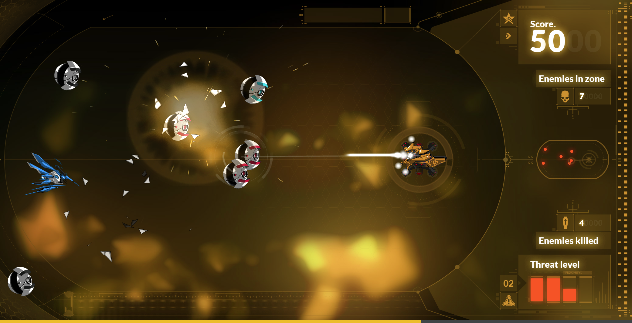You choose any language, including Python.

### Do you Like Books?

The community have lots of free books to download.

I liked Hitchhicker’s Guide to Python
While you aren’t children, I liked Python for Kids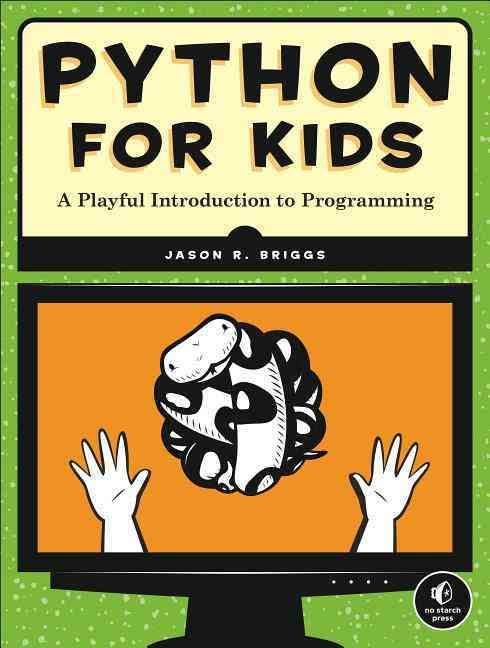### Learn with an Environment

At some point, you will need to develop your programs.

Might as well get your computer ready while learning.

## Functional Python

Let’s give you Part 1 of learning to program in Python.

Here we will quickly cover the basics.

Don’t worry if you miss or don’t understand, as you are learning online, right?

### Trying Some Thing

Try anything out by opening the Terminal program (called PowerShell in Windows), and typing:

```   python
```

Type any Python code you see:

```   >>> 3 * 10 + 3 + 7 / 2
51
```

Notice how it answers you.

### Comments

Some times you need to talk to your teammates through the code.

Words following `#` characters are comments are ignored.

```     # In this section, make sure we don't do anything that
# takes a long time, as this is run 50 times a second.
```

### Numbers

Python knows about numbers.

Treats integers and floating point numbers differently:

```     an_integer = 42
almost_pi = 3.141592653589793   # Notice the dot!
```

Floating points are approximations to real numbers.

### Math Operators

Basic math operations:

• `+` : addition
• `-` : subtraction
• `*` : multiplication
• `/` : integer division, e.g. `10 / 3` is `3`
Note: `10 / 3.0` is `3.3333333333333335`
• `%` : modulus, e.g. `10 % 3` is 1
• `**` : exponents, e.g. `2 ** 3` is `8`

### Math Functions

All other math is done through functions:

```     >>> import math

>>> math.floor(3.8)
3.0
>>> math.ceil(3.8)
4.0
>>> math.sqrt(16)
4.0
>>> math.degrees( math.pi / 2)
90.0
>>> math.radians(90) == math.pi / 2
True
>>> math.sin( math.pi / 2 )
1.0
```

### Variables

Store things in variables:

```     a = 5
not_the_answer = 41
```

Don’t need a `var` label (Python just knows)

Read them out with just the variable name:

```     the_answer = 1 + not_the_answer
```

### Conditionals

Use double-equals to compare things:

```     >>> the_answer == 42
True
>>> not_the_answer == 42
False
```

Do things if `True`:

```     if statement is True:
# do_something here
elif other_statement is True:
# do something lese here
else:
# otherwise do this
```

### Indentation

Notice the colon character and the indention.

```     if the_answer == 42:
print "I have the answer"
print "But I won't tell you"
```

The `:` tells Python the following lines should be grouped together.

Each line that connects to the `:` must be indented 4 spaces.

Don’t use tabs!

### Interactive Indentation

If you run `python` without a program, how do multilines work?

```   >>> if a_number == 42:
... ▮
```

The `...` prompt waits for more lines.
Type a blank carriage return to end it:

```     >>> if a_number == 42:
...    "I have the answer!"
...
'I have the answer!'
>>> ▮
```

### Strings

Put words between matching quotes:

```     a_phrase = "Hey! There's a tree!"
another = 'She said, "Hello." I said nothing.'
```

Use three quotes for strings on many lines:

```     paragraph = """Nam euismod tellus id erat.  Etiam vel neque nec dui dignissim
bibendum.  Donec hendrerit tempor tellus.  Donec posuere augue in
quam."""
```

### Pass

The `pass` statement doesn’t do anything.

It is a placeholder for code yet to come.

Finished code never has `pass` statements.

### Structures to hold Data

Helpful to Group logical data together:

• Lists

```       ['orange', 'apple', 'pear', 'banana', 'kiwi', 'apple', 'banana']
```
• Tuples

```       (12345, 54321, 'hello!')
```
• Dictionaries

```       {'jack': 4098, 'sape': 4139}
```
• Sets

```     {'apple', 'orange', 'pear', 'banana'}
```

#### Lists

Lists are ordered things:

```      fruit = ['orange', 'apple', 'pear', 'banana', 'kiwi', 'apple', 'banana']
```

Things don’t have to be the same:

```       howard = ['Howard', 53, 'tofu']
```

Get an element by an index:

```      >>> howard     # The first index is 0
53
```

Call methods:

```      >>> fruit.count('banana')
2
```

#### Tuples

Tuples are like lists:

```       tup = (12345, 54321, 'hello!')
```

Used by reassigning:

```       >>> x, y, z = tup
>>> x
12345
>>> z
'hello!'
```

Lists can change:

```       >>> fruit = 'avocado'
```

Tuples don’t:

```       >>> tup = 5
Traceback (most recent call last):
File "<input>", line 1, in <module>
tup = 5
TypeError: 'tuple' object does not support item assignment
```

#### Dictionaries

A dictionary (also called a hash or hashmap) indexed by a key:

```       >>> points = {'jack': 4098, 'sape': 4139}
>>> points['jack']
4098
```

Has functions too:

```       >>> points.values()
[4139, 4098]
>>> points.keys()
['sape', 'jack']
```

#### Sets

A set is list a list, but can’t have duplicates:

```      >>> fruit = {'apple', 'orange', 'apple', 'pear', 'orange', 'banana'}
>>> len(fruit)     # Tells us how many are in a list or set
4
>>> fruit
set(['orange', 'pear', 'apple', 'banana'])
```

### Functions

Put re-useable code in a function:

```     def greeting():
return 'Hello, how are you?'
```

Remember the `return` statement.

Call it with parenthesis:

```   >>> greeting()
'Hello, how are you?'
```

#### Parameters

Functions normally take parameters:

```     def square(num):
return num * num
```

Call it with whatever parameters it requires:

```   >>> square(5)
25
```

#### Function Assignment

Let’s have an increment function:

```      def inc(num):
return num + 1
```

Typically we use the values from a function:

```      >>> x = 5          # Creates a new variable
>>> x = inc(x)     # Reassigns that variable
>>> x = inc(x)
>>> x              # Show us the contents:
7
```

#### Default Values

A function can have optional parameters:

```      def inc(num = 0):
return num + 1
```

Here, you can call it with, or without a number:

```      >>> inc(5)
6
>>> inc()
1
```

#### None is a Type

Something can be nothing, called `None`:

```      def greeting(name = None):
if name:
return 'Hello, ' + name + ', how are you?'
else:
return 'Hello, how are you?'
```

Notice we don’t say: `if name == None:`

```      >>> greeting('Howard')
'Hello, Howard, how are you?'
>>> greeting()
'Hello, how are you?'
```

### Documentation

Each function should describe what it does:

```     def function_name():
'''String that describes what the function does'''
pass
```

View the function’s description and parameters:

```     >>> help(function_name)
```

Displays:

```     Help on function function_name in module __console__:

function_name()
String that describes what the function does
```

### Are we done?

Whew!### What have we Learned?

• Type `python` to try it out
• Pay attention to indentation
• Basic variable types: integers, floats, strings and booleans … and `None`
• Basic data groupings: lists, tuples, dictionaries, and sets
• Creating and calling functions

### Summary

• Fast, fast overview of Python.
• Have not learned Python, yet.
• You need to review and practice this stuff.
• Questions??

## Classes in Python

### Reviewing Variables

Create variables with a name and assignment:

```     foobar = 56
```

You can change its value with re-assignment:

```     foobar = "Hello there"
```

### Thought Experiment

Pretend we need to draw four robots:

What information do we need for each robot?

### Thought Experiment

What information do we need for each robot?

• X coordinate
• Y coordinate
• Directional angle
• Color

### Problem with Naming

Since we have more than one robot, we could:

• `red_rover_robot_x`
• `red_rover_robot_y`
• `red_rover_robot_direction`
• `red_rover_robot_color`
• `blue_glue_robot_x`
• `blue_glue_robot_y`
• `blue_glue_robot_direction`

Ugh.

### Using a Dictionary

Do you remember dictionaries?

Do you know when they are used?

### Using a Dictionary

A dictionary could easily group these:

```     red_robot = {
'x': 100,
'y': 240,
'direction': -24,
'color': 'red'
}
```

Get the `x` coordinate with:

```     red_robot['x']  # Returns 100
```

Change its values too:

```     red_robot['x'] = 50
```

### Making a Class

A nicer way is to define a new type:

```     class Robot:
x = 0
y = 0
direction = 0
color = ''
```

Notice the variables must have a value.
Typically start with `0` or empty strings.

### Making a Class

A class is like a generic, boring thing:

A `class` describes an initial state.

### Making a Class and Object

But we use the class to make an object instance:

```     blue_bell = Robot()
```

Looks like a function call, right?

### Changing an Object

Once the object is made, we often change it like a dictionary:

```     blue_bell = Robot()
blue_bell.x = 74
blue_bell.y = -12
blue_bell.direction = -37
```

Dots are used to separate the object from one of its parts.

### Reading an Object’s State

With our `blue_bell` variable, we can read its values:

```     blue_bell.x      # Returns 74
blue_bell.color  # Returns 'blue'
```

### Changing an object

We could change anything allowed to change, even the color:

```     blue_bell.color = 'red'
```

### Making a Class and Objects

We can use that class to make lots of objects:

We can then make robot instances (objects):

```     blue_bell = Robot()
red_rover = Robot()
mellow_yellow = Robot()
```

### Changing Objects … wrong way

Let’s turn our robot:

```   def turn(robot, amount):
robot.direction = robot.direction + amount
```

This means that our robot function needs to know a lot about a robot’s inside variables.

### Changing Objects … right way

Better to put the `turn` function inside the class:

```     class Robot:
x = 0
y = 0
direction = 0
color = ''

def turn(robot, amount):
robot.direction = robot.direction + amount
```

Calling the `turn` function when inside the class looks like:

```     blue_bell.turn(36)
```

Does this look wrong to you? Why?

### Inside Functions are called Methods

By tradition, the first parameter to a method is named `self`:

```     class Robot:
# ... fields ...

def turn(self, amount):
self.direction = self.direction + amount
```

### Changing a Python Object

Let’s make an object:

```     mr_roboto = Robot()
```

Note, `mr_roboto.direction` begins with `0`. What if:

```     mr_roboto.turn(45)
```

What will `mr_roboto.direction` be?

Now, call:

```     mr_roboto.turn(-10)
```

What will `mr_roboto.direction` be?

### Initializing a Python Object

What if we didn’t want our robot’s `x` and `y` to be `0`?

```     class Robot:
x = 0
y = 0

def __init__(self, start_x, start_y):
self.x = start_x
self.y = start_y
```

Look carefully at this code. Do you understand it?

```     miss_rob = Robot(50, 20)
```

### Why Self?

Same version different internal names:

```     class Robot:
x = 0
y = 0

def __init__(self, x, y):
self.x = x
self.y = y
```

Why does this work? What does `x` refer?

### Init is Very Special

Does this work?

```     class Robot:
def __init__(self, x, y):
self.x = x
self.y = y
```

Any variables set on `self` become fields.

### Default Init Parameters

Another way to set default values for `x` and `y`:

```     class Robot:
def __init__(self, x = 0, y = 0):
self.x = x
self.y = y
```

Both of these work:

```     red_rover = Robot()         # Start at 0,0
blue_bot  = Robot(50, 50)   # Start at 50,50
```

### Drawing Function

Let’s create a Robot drawing function:

```     def draw(self):
turtle.setx( self.x )
turtle.sety( self.y )
# Or turtle.goto( self.x, self.y )
turtle.setheading( self.direction )
turtle.color( self.color )
# ...
```

### What about Drawing

What if each robot looked different:

### What about Drawing

What if each robot looked different:

We could create functions:

• `def draw_two_wheel_robot():`
• `def draw_four_wheel_robot():`
• `def draw_tread_robot():`

Ugh.

### Another Example

Let’s plug a wire from a motor controller pin to a motor…

```     class Motor:
def __init__(self, pin):
self.pin = pin

def power(self, voltage):
if not voltage in (-1, 0, 1):
raise Exception("Voltage is +/-1 or 0 for power")
# Send voltage value to self.pin ...
```

We can use it like:

```     left_motor = Motor(1)
right_motor = Motor(2)

# Turn to the right!
left_motor.power(-1)
right_motor.power(1)
```

### Classes using Classes

One class can take advantage of another.

Sends power to motor on some pin:

```     class TankRobot:
left_motor = Motor(1)      # Control motor on pin 1
right_motor = Motor(2)     # Control motor on pin 2

def forward(self):
self.left_motor.power(1)
self.right_motor.power(1)

def left(self):
self.left_motor.power(1)
self.right_motor.power(-1)

def right():
self.left_motor.power(-1)
self.right_motor.power(1)
```

### The Case for Classes

What if …

We wanted `Robot` to be either drive train type…

• Tank Drivetrain:
• Pin 1 controls left motor
• Pin 2 controls right motor
• Swerve Drivetain:
• Pin 1 controls direction of all 4 motors
• Pin 2 controls pulse of all 4 motors

### The Case for Classes: Code

Modeling the Swerve Drive as `SwerveRobot`:

```     class SwerveRobot:
turner = Motor(1)      # Control motors on pin 1
wheels = Motor(2)      # Control motors on pin 2

def forward(self):
self.wheels.power(1)

def left(self):
for t in range(90):   # Pulse power 90 times for 90 degrees:
self.turner.power(-1)

def right():
for t in range(90):
self.turner.power(1)
```

Notice this example for a swerve drive train behaves like the tank drivetrain.

### Don’t Repeat Yourself (DRY)

Perhaps both `TankRobot` and `SwerveRobot` has same code:

```     class SwerveRobot:
def __init__(self, name):
self.name = name
# ... the rest is the same ...

class TankRobot:
def __init__(self, name):
self.name = name
# ... the rest is the same ...
```

Never duplicate code! Why not?

### Reusing Code with Inheritance

One approach: place duplicate code is parent class:

```     class Robot:
def __init__(self, name):
self.name = name

class SwerveRobot(Robot):
# ... the rest is the same ...

class TankRobot(Robot):
# ... the rest is the same ...

thomas = TankRobot("Thomas")
print(thomas.name) # Return 'Thomas'
```

## Testing Python

Date: 2017 Aug 18

Created: 2020-12-23 Wed 10:14